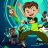# Hide Relative Average Volume Standard Deviation

X

#### XeoNoX

##### New member
This is the built in code of TOS for RelativeVolumeStDev

i however when its charted on a chart i just want to hide (not show) the bars that are under 2.0 times the relative volume.
example pic of what im trying to hide attached

Code:
``````#
# TD Ameritrade IP Company, Inc. (c) 2014-2019
#

declare lower;
declare zerobase;

input length = 60;
input numDev = 2.0;
input allowNegativeValues = no;

def rawRelVol = (volume - Average(volume, length)) / StDev(volume, length);
plot RelVol = if allowNegativeValues then rawRelVol else Max(0, rawRelVol);
plot StDevLevel = numDev;

RelVol.SetPaintingStrategy(PaintingStrategy.HISTOGRAM);
RelVol.SetLineWeight(3);
RelVol.DefineColor("Above", GetColor(0));
RelVol.DefineColor("Below", GetColor(2));
RelVol.AssignValueColor(if RelVol >= numDev then RelVol.Color("Above") else RelVol.Color("Below"));
StDevLevel.SetDefaultColor(GetColor(7));
StDevLevel.SetStyle(Curve.SHORT_DASH);``````T

#### tomsk

##### Well-known member
VIP
Warehouse
@XeoNoX Per your request I have modified your study so that it only displays bars ABOVE the dotted line.
Here is the code, I tested against your 1 min chart of AMD

Code:
``````declare lower;
declare zerobase;

input length = 60;
input numDev = 2.0;
input allowNegativeValues = no;

def rawRelVol = (volume - Average(volume, length)) / StDev(volume, length);
def RelVol = if allowNegativeValues then rawRelVol else Max(0, rawRelVol);

plot StDevLevel = numDev;
plot RV = if RelVol > numDev then RelVol else Double.NaN;

RV.SetPaintingStrategy(PaintingStrategy.HISTOGRAM);
RV.SetLineWeight(3);
RV.DefineColor("Above", GetColor(0));
RV.DefineColor("Below", GetColor(2));
RV.AssignValueColor(if RelVol >= numDev then RV.Color("Above") else RV.Color("Below"));
StDevLevel.SetDefaultColor(GetColor(7));
StDevLevel.SetStyle(Curve.SHORT_DASH);
# End Code``````

Last edited:
•XeoNoX and blakecmathis
X

#### XeoNoX

##### New member
cheers, thanks brotherCustom 1Custom 6